## Thursday, October 27, 2016

### 1D Haar Wavelet Down-Up Spikes

Introduction

HWTs are used to detect significant changes in signal values. In a previous post, I discussed 1D Haar Wavelet up-down spikes that can be used to detect changes in signals. Specifically, four types of spikes were proposed: up-down triangle, up-down trapezoid, down-up triangle, and down-up trapezoid. The difference between up-down and down-up spikes is the relative positions of the climb and decline segments. In trapezoid spikes, flat segments are always in between the climb and decline segments, regardless of their relative positions. In this post, I will define down-up spikes.

Dow-Up Spikes

Fig. 1 shows down-up triangle and trapezoid spikes.  In this figure, the lower graphs represent the possible values of the corresponding Haar wavelets at a chosen scale k.
The decline segment of the spike is characterized by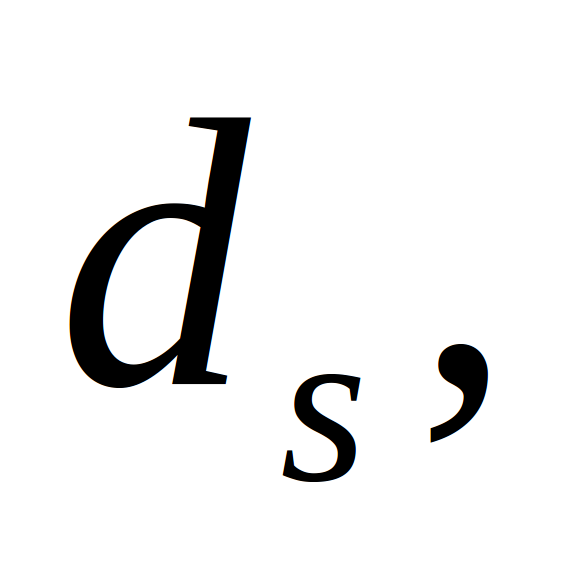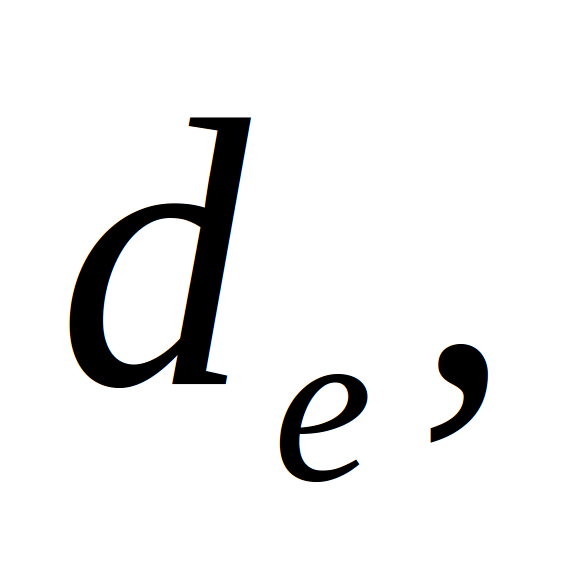andwhere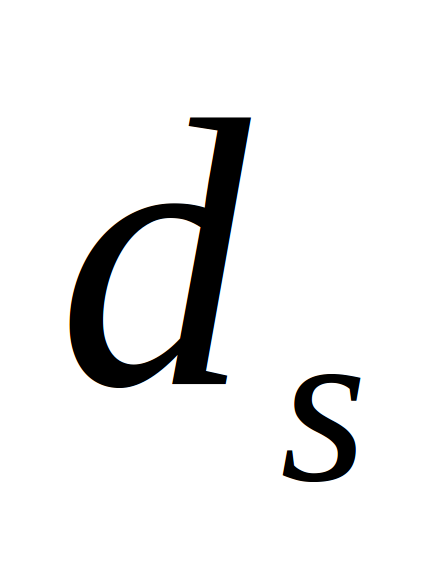and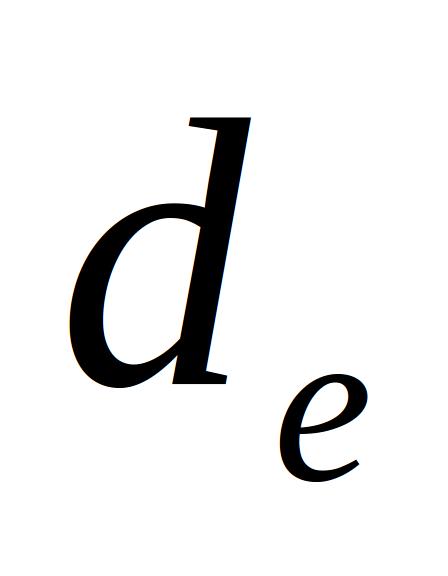are the abscissae of the beginning and end of the spike’s decline segment, respectively, when the wavelet coefficients decrease. If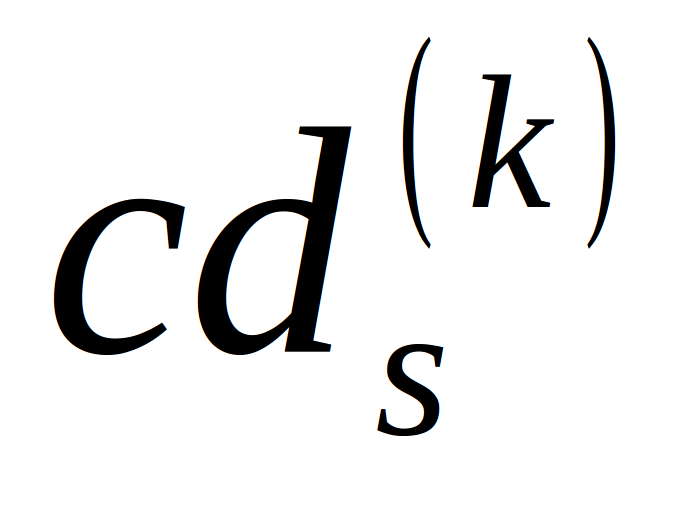and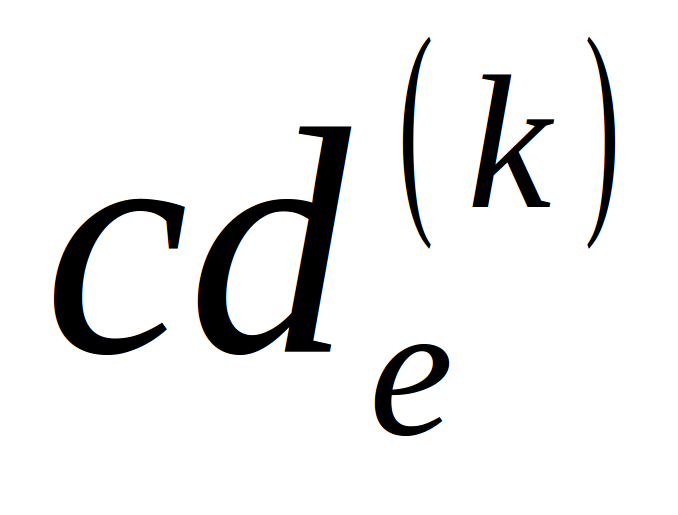are the k-th scale wavelet coefficient ordinates atandrespectively.
The values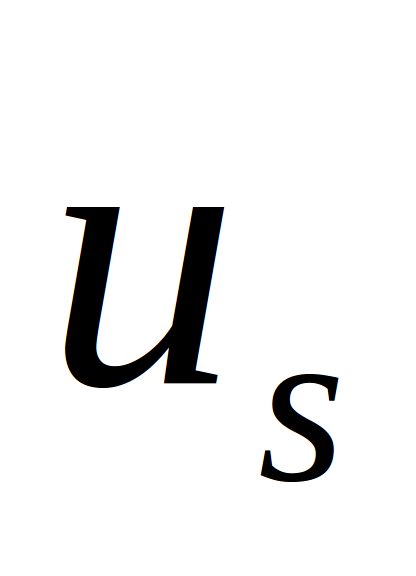and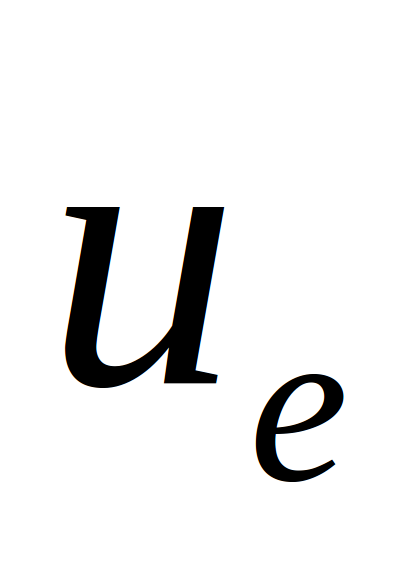are the abscissae of the beginning and end of the spike’s climb segment, respectively, when the wavelet coefficients of the 1D HWT increase;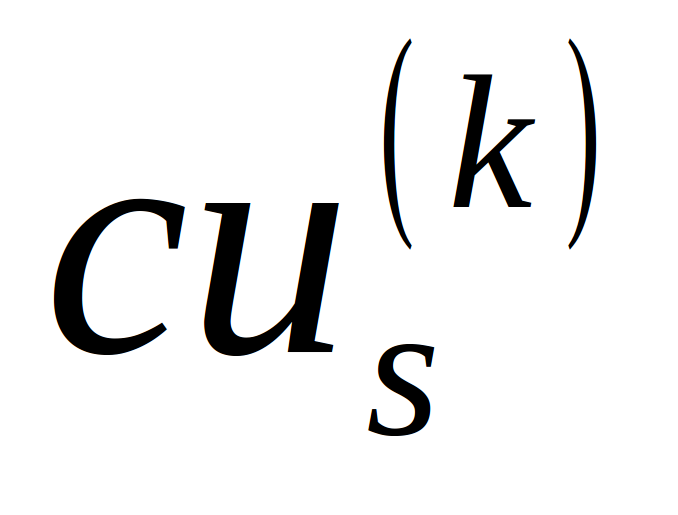and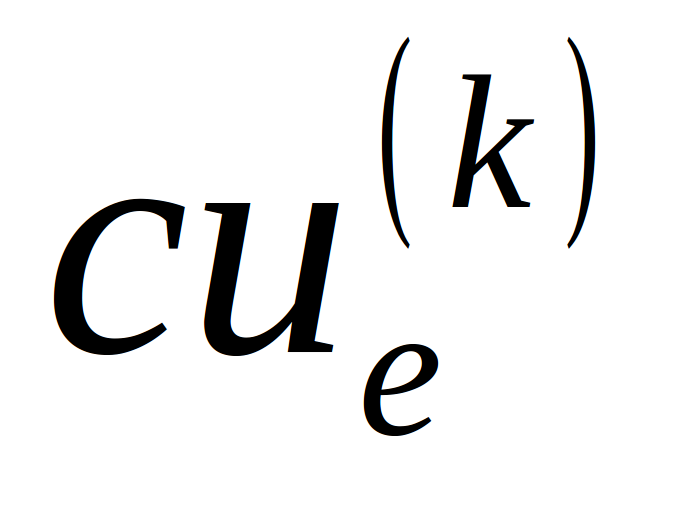are the k-th scale wavelet coefficient ordinates atand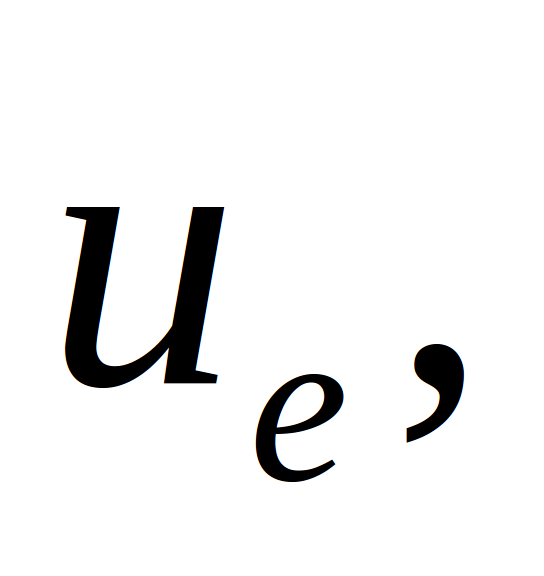respectively.
For a trapezoid down-up spike, the flat segment is characterized by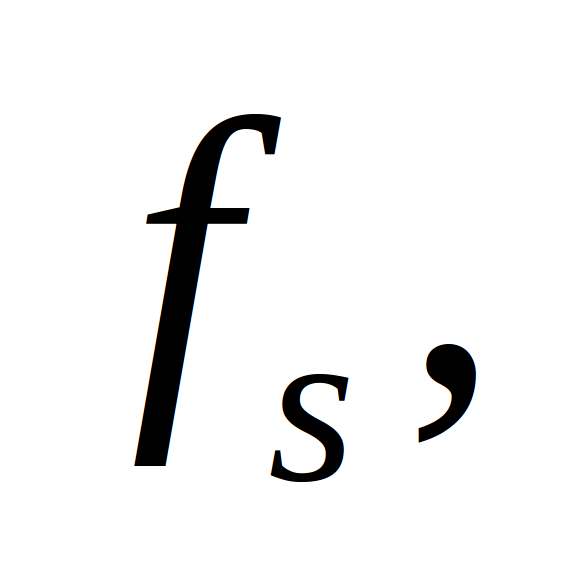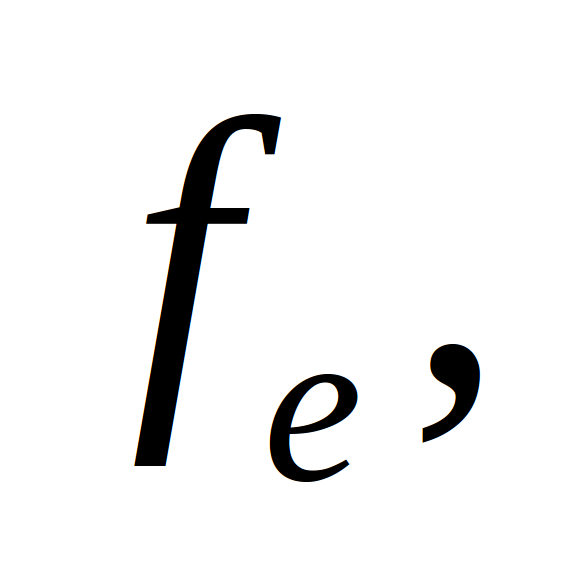and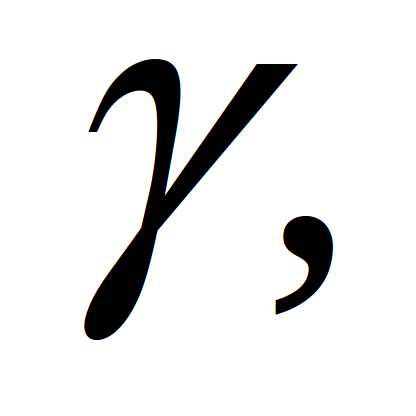where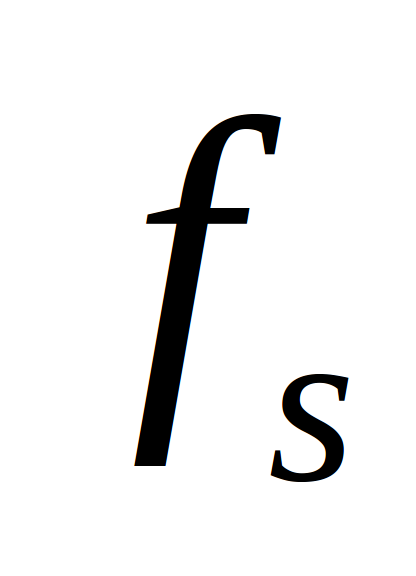and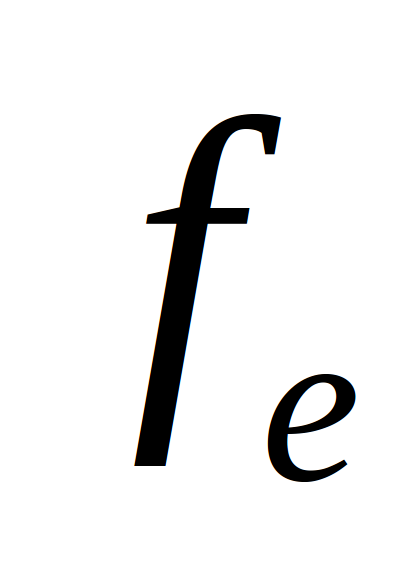are the abscissae of the beginning and end of the spike’s flat segment, respectively, over which the wavelet coefficients either remain at the same ordinate or have minor ordinate fluctuations. The values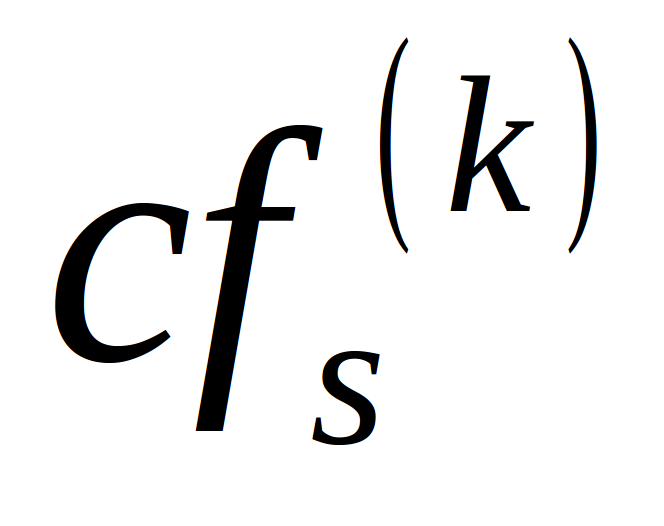andare the k-th scale wavelet coefficients corresponding toandrespectively.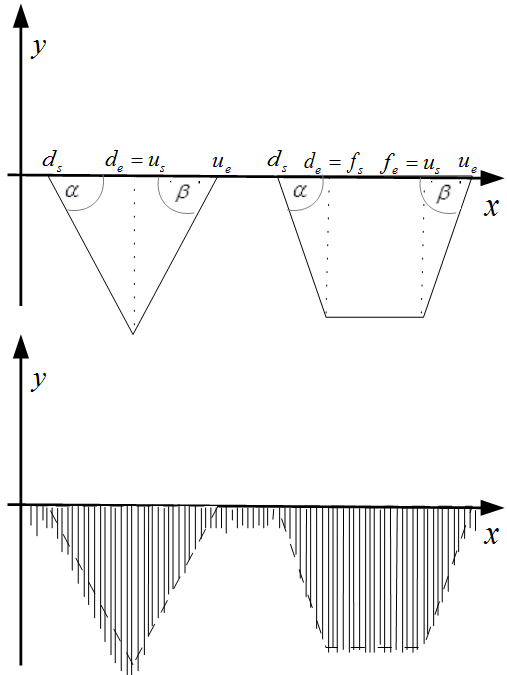Fig. 1. 1D Haar wavelet down-up spikes

A down-up spike describe signals that first decrease and then, after an optional flat segment, increase, unlike an up-down spike where a decline segment follows a climb segment. The climb and decline angles are measured in the same way as for up-down spikes.